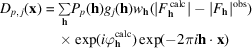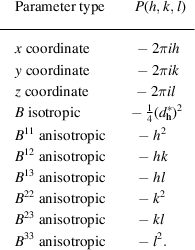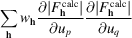International
Tables for
Crystallography
Volume B
Reciprocal space
Edited by U. Shmueli

International Tables for Crystallography (2010). Vol. B, ch. 1.3, pp. 97-98   | 1 | 2 |

## Section 1.3.4.4.7.5. Cruickshank's modified Fourier method

G. Bricognea

aGlobal Phasing Ltd, Sheraton House, Suites 14–16, Castle Park, Cambridge CB3 0AX, England, and LURE, Bâtiment 209D, Université Paris-Sud, 91405 Orsay, France

#### 1.3.4.4.7.5. Cruickshank's modified Fourier method

| top | pdf |

Cruickshank consolidated and extended Cochran's derivations in a series of classic papers (Cruickshank, 1949b, 1950, 1952, 1956). He was able to show that all the coefficients involved in the right-hand side and normal matrix of the least-squares method could be calculated by means of suitable differential Fourier syntheses even when the atoms overlap. This remarkable achievement lay essentially dormant until its independent rediscovery by Agarwal in 1978 (Section 1.3.4.4.7.6).

To ensure rigorous equivalence between the summations over(in the expressions of least-squares right-hand side and normal matrix elements) and genuine Fourier summations, multiplicity-corrected weights were introduced by:where Gh denotes the orbit of h andits isotropy subgroup (Section 1.3.4.2.2.5). Similarly, derivatives with respect to parameters of symmetry-unique atoms were expressed, via the chain rule, as sums over the orbits of these atoms.

Letbe the label of a parameterbelonging to atoms with label j. Then Cruickshank showed that the pth element of the right-hand side of the normal equations can be obtained as, whereis a differential synthesis of the formwitha polynomial in (h, k, l) depending on the type of parameter p. The correspondence between parameter type and the associated polynomial extends Booth's original range of differential syntheses, and is recapitulated in the following table.Unlike Cochran's original heuristic argument, this result does not depend on the atoms being resolved.

Cruickshank (1952)also considered the elements of the normal matrix, of the formassociated with positional parameters. Theblock for parametersandmay be writtenwhich, using the identitybecomes(Friedel's symmetry makesredundant on the last line). Cruickshank argued that the first term would give a good approximation to the diagonal blocks of the normal matrix and to those off-diagonal blocks for whichandare close. On this basis he was able to justify the n-shift rule' of Shoemaker et al. (1950). Cruickshank gave this derivation in a general space group, but using a very terse notation which somewhat obscures it. Using the symmetrized trigonometric structure-factor kernelof Section 1.3.4.2.2.9and its multiplication formula, the above expression is seen to involve the values of a Fourier synthesis at points of the form.

Cruickshank (1956)showed that this analysis could also be applied to the refinement of temperature factors.

These two results made it possible to obtain all coefficients involved in the normal equations by looking up the values of certain differential Fourier syntheses ator at. At the time this did not confer any superiority over the standard form of the least-squares procedure, because the accurate computation of Fourier syntheses was an expensive operation. The modified Fourier method was used by Truter (1954)and by Ahmed & Cruickshank (1953a), and was incorporated into the program system described by Cruickshank et al. (1961). A more recent comparison with the least-squares method was made by Dietrich (1972).

There persisted, however, some confusion about the nature of the relationship between Fourier and least-squares methods, caused by the extra factorswhich make it necessary to compute a differential synthesis for each type of atom. This led Cruickshank to conclude that in spite of their remarkable similarities the least-squares and modified-Fourier methods are fundamentally distinct'.

### References

Ahmed, F. R. & Cruickshank, D. W. J. (1953a). A refinement of the crystal structure analysis of oxalic acid dihydrate. Acta Cryst. 6, 385–392.
Cruickshank, D. W. J. (1949b). The accuracy of atomic co-ordinates derived by least-squares or Fourier methods. Acta Cryst. 2, 154–157.
Cruickshank, D. W. J. (1950). The convergence of the least-squares and Fourier refinement methods. Acta Cryst. 3, 10–13.
Cruickshank, D. W. J. (1952). On the relations between Fourier and least-squares methods of structure determination. Acta Cryst. 5, 511–518.
Cruickshank, D. W. J. (1956). The determination of the anisotropic thermal motion of atoms in crystals. Acta Cryst. 9, 747–753.
Cruickshank, D. W. J., Pilling, D. E., Bujosa, A., Lovell, F. M. & Truter, M. R. (1961). Crystallographic calculations on the Ferranti Pegasus and mark I computers. In Computing Methods and the Phase Problem in X-Ray Crystal Analysis, edited by R. Pepinsky, J. M. Robertson & J. C. Speakman, pp. 32–78. Oxford: Pergamon Press.
Dietrich, H. (1972). A reconsideration of Fourier methods for the refinement of crystal structures. Acta Cryst. B28, 2807–2814.
Shoemaker, D. P., Donohue, J., Schomaker, V. & Corey, R. B. (1950). The crystal structure of LS-threonine. J. Am. Chem. Soc. 72, 2328–2349.
Truter, M. R. (1954). Refinement of a non-centrosymmetrical structure: sodium nitrite. Acta Cryst. 7, 73–77.